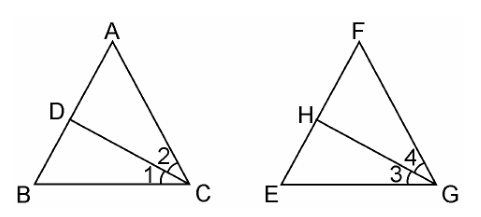Guru

# CD and GH are respectively the bisectors of ∠ACB and ∠EGF such that D and H lie on sides AB and FE of ΔABC and ΔEFG respectively. If ΔABC ~ ΔFEG, Show that: (i) CD/GH = AC/FG Q10.(1)

• 0

How i solve this tough question in easy way of chapter triangles of class 10th of exercise 6.3 of class 10th math, please help me to find out the solution of this question  CD and GH are respectively the bisectors of ∠ACB and ∠EGF such that D and H lie on sides AB and FE of ΔABC and ΔEFG respectively. If ΔABC ~ ΔFEG, Show that: (i) CD/GH = AC/FG

Share

1.(i) From the given condition,

ΔABC ~ ΔFEG.

∴ ∠A = ∠F, ∠B = ∠E, and ∠ACB = ∠FGE

Since, ∠ACB = ∠FGE

∴ ∠ACD = ∠FGH (Angle bisector)

And, ∠DCB = ∠HGE (Angle bisector)

In ΔACD and ΔFGH,

∠A = ∠F

∠ACD = ∠FGH

∴ ΔACD ~ ΔFGH (AA similarity criterion)

⇒CD/GH = AC/FG

• 0